# Create a random mixture¶

import openturns as ot
import openturns.viewer as viewer
from matplotlib import pylab as plt

ot.Log.Show(ot.Log.NONE)


## Create a mixture of distributions¶

We define an affine combination of input random variables.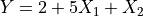where:

•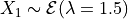•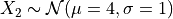This notion is different from the Mixture where the combination is made on the probability density functions and not on the univariate random variable.

We create the distributions associated to the input random variables :

X1 = ot.Exponential(1.5)
X2 = ot.Normal(4.0, 1.0)


We define an offset a0 :

a0 = 2.0


We create the weights :

weight = [5.0, 1.0]


We create the affine combination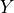:

distribution = ot.RandomMixture([X1, X2], weight, a0)
print(distribution)

RandomMixture(Normal(mu = 6, sigma = 1) + Exponential(lambda = 0.3, gamma = 0))


We get its mean :

mean = distribution.getMean()
print("Mean : %.3f" % mean)

Mean : 9.333


its variance :

variance = distribution.getCovariance()[0, 0]
print("Variance : %.3f" % variance)

Variance : 12.111


the 90% quantile :

quantile = distribution.computeQuantile(0.9)
print("0.9-quantile : %.3f" % quantile)

0.9-quantile : 13.825


We can get the probability of therandom variable to exceed 10.0 :

prb = distribution.computeSurvivalFunction(10.0)
print("Probability : %.3f" % prb)

Probability : 0.315


We draw its PDF :

graph = distribution.drawPDF()
view = viewer.View(graph)We draw its CDF :

graph = distribution.drawCDF()
view = viewer.View(graph)## Create a discrete mixture¶

In this paragraph we build the distribution of the value of the sum of 20 dice rolls.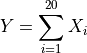where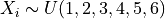We create the distribution associated to the dice roll :

X = ot.UserDefined([[i] for i in range(1, 7)])


Let’s roll the dice a few times !

sample = X.getSample(10)
print(sample)

    [ v0 ]
0 : [ 1  ]
1 : [ 1  ]
2 : [ 6  ]
3 : [ 2  ]
4 : [ 4  ]
5 : [ 2  ]
6 : [ 3  ]
7 : [ 1  ]
8 : [ 3  ]
9 : [ 2  ]

N = 20


We create a collection of identically distributed Xi :

coll = [X] * N


We create the weights and an affine combination :

weight = [1.0] * N
distribution = ot.RandomMixture(coll, weight)


We compute the probability to exceed a sum of 100 after 20 dice rolls :

print("Probability : %.3g" % distribution.computeComplementaryCDF(100))

Probability : 1.58e-05


We draw its PDF :

graph = distribution.drawPDF()
view = viewer.View(graph)and its CDF :

graph = distribution.drawCDF()
view = viewer.View(graph)Display all figures

plt.show()